# B4J Code Snippet[BANano]: Sharing the goodness

#### Mashiane

##### Expert
BANanoSQL: Query an object / map based list
BANanoQueryJSON was added to BANano which serves a better method of applying these types of queries!

Ta!

#### Mashiane

##### Expert
So I used the word "typeOf" as a map key value in my app: DON'T.

#### Mashiane

##### Expert
BANanoSQL: Query a JSON array quicky.

B4X:
``````'convert json string to list
Dim fldJSON as List = BANano.FromJSON(strJson)
'open the database
db.OpenWait("bvmdesigner", "bvmdesigner")
'execute the query
Dim rslt As List = db.ExecuteWait("select * from ? where colactive = 'Yes'", Array(fldJSON))
Log(rslt)``````

•joulongleu and Johan Hormaza

#### Mashiane

##### Expert
Warp Drive

When I saw this I was just mind blown, realizing the power of css and html. Wow

#### Attachments

• 7.4 KB Views: 7

#### joulongleu

##### Active Member
Warp Drive

When I saw this I was just mind blown, realizing the power of css and html. Wow

Very good, can join BANanoVueMaterial?

•Mashiane and micro

#### Mashiane

##### Expert

This happens when at times you compile a project.

Ta!

•joulongleu

#### Mashiane

##### Expert
I wanted to change the App Document Title on Demand i.e. Browser Tab Title.

Watch BANanoJavascriptDemo changing to Anele Mbanga (Mashy) on the browser when the document is clicked.B4X:
``````document = banano.Window.GetField("document")
document.SetField("title", "Anele Mbanga (Mashy)")``````
Ta!

•Johan Hormaza and alwaysbusy

#### Mashiane

##### Expert
1. Fire a click event on a checkbox via code.
2. Toggle the direction of text from LRT (left to right) to RTL (right to left)

NB: We have made the code to look like javascript in this case..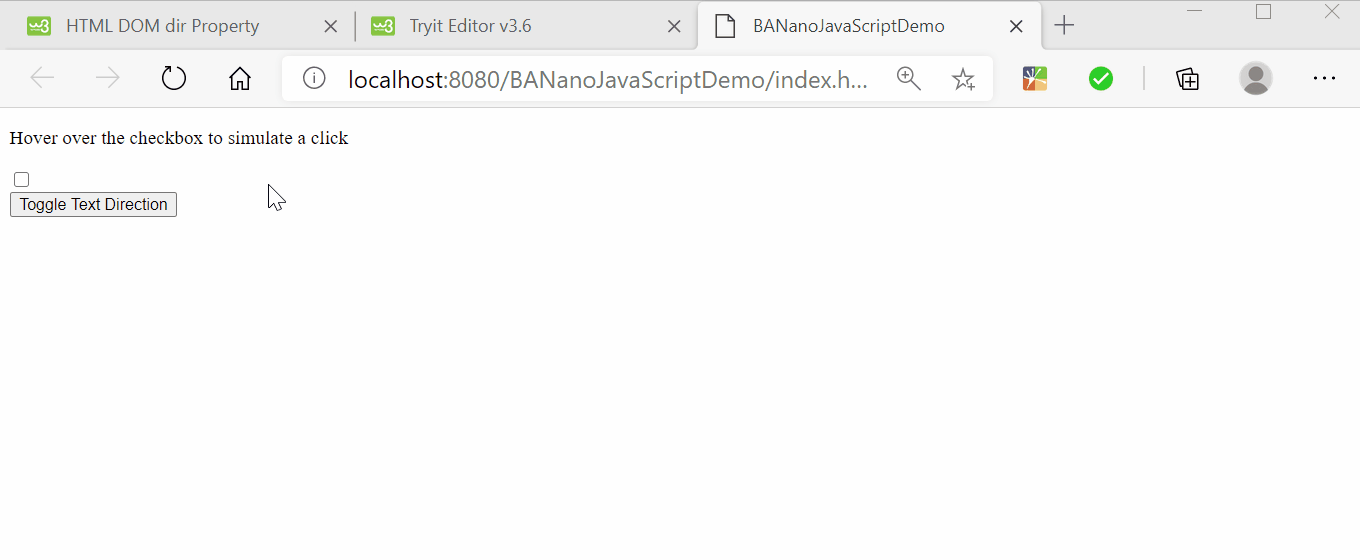1. Create a form. add an input checkbox. Assign mouseover event. Assign a click event.
2. On the mouseover event, fire a click event.

B4X:
``````'create a form
Dim f As JSElement = document.createElement("FORM")
'create a checkbox
Dim i As JSElement = document.createElement("INPUT")
i.setAttribute("type", "checkbox")
i.ID = "mycheck"
f.appendchild(i)
document.body.appendChild(f)

Sub hoverit(e As BANanoEvent)
document.getElementById("mycheck").click
End Sub``````
This is the click event

B4X:
``````'fire a click event
Sub click
Element.RunMethod("click", Null)
End Sub``````
For the toggle, we are setting the dir attribute of the paragraph from LRT to RTL.

We add the button to run the toggle

B4X:
``````Dim btnTD As JSElement = document.createElement("BUTTON")
btnTD.appendChild(document.createTextNode("Toggle Text Direction"))
document.body.appendChild(btnTD)``````
When the button is clicked, we check the direction and then change it

B4X:
``````Sub toggledir(e As BANanoEvent)
Dim dir As String = document.getElementById("note").dir
Select Case dir
Case document.DIR_LRT, ""
document.getElementById("note").dir = document.DIR_RTL
Case document.DIR_RTL
document.getElementById("note").dir = document.DIR_LRT
End Select
End Sub``````
Where the paragraph is:

B4X:
``````'add a paragraph
Dim p As JSElement = document.createElement("P")
p.ID = "note"
p.appendChild(document.createTextNode("Hover over the checkbox to simulate a click"))
document.body.append(p)``````

•joulongleu and Johan Hormaza

#### Mashiane

##### Expert
Changing images at runtime.

We have the js equivalent and the banano equivalent.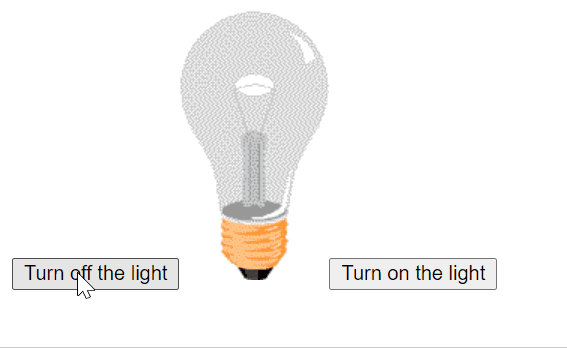B4X:
``````Sub turnon(e As BANanoEvent)
'document.getElementById("bulb").src = "./assets/pic_bulbon.gif"
BANano.GetElement("#bulb").SetAttr("src", "./assets/pic_bulbon.gif")
End Sub

Sub turnoff(e As BANanoEvent)
'document.getElementById("bulb").src = "./assets/pic_bulboff.gif"
BANano.GetElement("#bulb").SetAttr("src", "./assets/pic_bulboff.gif")
End Sub``````
Ta!

•Erel and joulongleu

#### Mashiane

##### Expert
Javascript Arrays

Created a simple class to handle arrays: this extends some B4X list functionalities.

Example 1 - forEach

B4X:
``````Dim a1 As JSArray
a1.Initialize(Array As String("Anele", "Mbanga", "(Mashy)"))
Log("a1 size: " & a1.size)
text = "<ul>"
a1.foreach(Me, "buildlist")
text = text & "</ul>"
'
Log(text)``````
1. Create an array with some simple items, get the size of the array. For each item in the array, we run the forEach array method passing it a callback.

B4X:
``````Sub buildlist(value As Object)
text = text & "<li>" & value & "</li>"
End Sub``````
What this does is to receive each value in the array, build but a <li></li> and the output is.

B4X:
``<ul><li>Anele</li><li>Mbanga</li><li>(Mashy)</li></ul>``
Example 2: JS - every

Every checks if all values in an array meets a condition, if they all do, a True is returned / False

B4X:
``````'every
Dim ages As JSArray
ages.Initialize(Array As Int(32, 33, 16, 40))

'check if all items are >= 18
Dim sout As Boolean = ages.every(Me, "checkadult")
Log("every: " & sout)``````
The callback is

B4X:
``````Sub checkadult(age As Int) As Boolean
age = banano.parseint(age)
Return age >= 18
End Sub``````
This method will return false because one of the array items is less than 18.

Example 3: filter

Filter returns the items in the array meeting the callback

B4X:
``````'return all items >= 18
Dim xout As JSArray = ages.filter(Me, "checkadult")
Log("filter: " & xout.items)``````
output of this is:

B4X:
``filter: 32,33,40``
This has removed 18 as it does not meet the condition.

Ta!

NB: Class to be provided - testing

Last edited:
•joulongleu

#### alwaysbusy

##### Expert
1. Fire a click event on a checkbox via code.
2. Toggle the direction of text from LRT (left to right) to RTL (right to left)

NB: We have made the code to look like javascript in this case..

View attachment 100307
Note that the pure BANano equivalent would be:
B4X:
``````Sub BANano_Ready()
Dim body As BANanoElement
body.Initialize("#body")

Dim form As BANanoElement
form.Initialize("<form>") 'make a new form tag

Dim input As BANanoElement
input.Initialize("<input>") 'make a new input tag
input.SetAttr("id", "mycheck")
input.SetAttr("type", "checkbox")
input.On("mouseover", Me, "hoverit")
input.On("click", Me, "clickit")
form.Append(input)
body.Append(form)

Dim btnTD As BANanoElement
btnTD.Initialize("<button>") ' make a new button tag
btnTD.SetText("Toggle Text Direction")
btnTD.On("click", Me, "Toggledir")
body.Append(btnTD)

Dim p As BANanoElement
p.Initialize("<p>") ' make a new p tag
p.SetAttr("id", "note")
p.Settext("Hover over the checkbox to simulate a click")
body.Append(p)
End Sub

Sub HoverIt(e As BANanoEvent) 'ignore
BANano.GetElement("#mycheck").Trigger("click", Null)
End Sub

Sub Clickit(e As BANanoEvent) 'ignore
End Sub

Sub ToggleDir(e As BANanoEvent) 'ignore
Dim Note As BANanoElement = BANano.GetElement("#note")
Dim dir As String = Note.GetAttr("dir")
Select Case dir
Case "rtl"
Note.SetAttr("dir", "")
Case Else
Note.SetAttr("dir", "rtl")
End Select
End Sub``````
I will change the param in Append/Prepend/After/Before to an Object as this doesn't seem obvious that one can also use another BANanoElement as a parameter.

Alwaysbusy

•joulongleu and Mashiane

#### Mashiane

##### Expert
Note that the pure BANano equivalent would be:

I will make the param in Append/Prepend/After/Before to an Object as this doesn't seem obvious that one can also use another BANanoElement as a parameter.
Sounds perfect.

•joulongleu

#### Mashiane

##### Expert
Doing a little bit of maths

NB - this has some advanced stuff using the BANanoObject, however the math operations are just B4X you already know stuff.

Some of javascript math statements are rather odd. For example for the b4x Mod, javascript uses %. When learning javascript and want to port some javascript apps to BANAno, you might be faced with understanding what all this means.

B4X has handy math functions, its just the understand of what ++a; or a *= 5; of javascript, means at times when you encounter such.
By the way ++1 means a = a + 1 whilst a *=5 means a = a * 5.

Anyway for this we will use BANanoObject, this will help us open our document, write some stuff to it. We will only create a <br> element, however for this we will use the document.write method to do so. So using document.open, document.write and document.close, we do some math and show it on our page.

NB: Document.Open clears everything on the page including any given page title.

1. get the document object from the window object. The window object is the parent element for all page element. This is the BANanoWindow object.

B4X:
``Dim document As BANanoObject = banano.Window.GetField("document")``
2. Because the js in BANAno is loaded async, we need to open the document
B4X:
``document.RunMethod("open", Null)``
We will close it later with

B4X:
``document.RunMethod("close", Null)``
After we finish writing to it

3. Let's do the Math. First a helper function

For this we need to write some stuff to the document. For this let's define a shortcut

B4X:
``````Sub write(value as string)
document.RunMethod("write", array(value))
End Sub``````
4. The output...B4X:
``````    Dim a As Int = 33
Dim b As Int = 10
Dim c As String = "Test"
Dim linebreak As String = "<br />"
Dim result As Int = 0
'
write("a = ")
write(a)
write(linebreak)
'
write("b = ")
write(b)
write(linebreak)
'
write("c = ")
write(c)
write(linebreak)

write("a + b = ")
result = a + b
write(result)
write(linebreak)

write("a - b = ")
result = a - b
write(result)
write(linebreak)

write("a / b = ")
result = a / b
write(result)
write(linebreak)

write("a % b (i.e Mod) = ")
result = a Mod b
write(result)
write(linebreak)

write("a + b + c = ")
result = a + b + c
write(result)
write(linebreak)

a = a + 1
write("++a (i.e a = a + 1) = ")
result = a
write(result)
write(linebreak)
'
a = a + 5
write("a += 5 (i.e a = a + 5) = ")
result = a
write(result)
write(linebreak)
'
a = a - 5
write("a -= 5 (i.e a = a - 5) = ")
result = a
write(result)
write(linebreak)
'
a = a * 2
write("a *= 2 (i.e a = a * 2) = ")
result = a
write(result)
write(linebreak)
'
a = a / 2
write("a /= 2 (i.e a = a / 2) = ")
result = a
write(result)
write(linebreak)
'
a = a Mod 2
write("a %= 2 (i.e a = a Mod 2) = ")
result = a
write(result)
write(linebreak)

b = b - 1
write("--b (i.e b = b - 1 = ")
result = b
write(result)
write(linebreak)

'close the document``````
Ta!

#### Mashiane

##### Expert
Math Comparisons (continuation from previous...)

Output..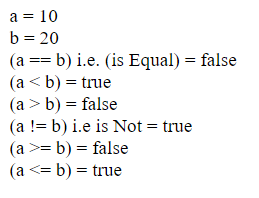B4X:
``````Dim a As Int = 10
Dim b As Int = 20
Dim linebreak As String = "<br />"
Dim result As Object

write("a = ")
write(a)
write(linebreak)
'
write("b = ")
write(b)
write(linebreak)

write("(a == b) i.e. (is Equal) = ")
result = (a = b)
write(result)
write(linebreak)

write("(a < b) = ")
result = (a < b)
write(result)
write(linebreak)

write("(a > b) = ")
result = (a > b)
write(result)
write(linebreak)

write("(a != b) i.e is Not = ")
result = (a <> b)
write(result)
write(linebreak)

write("(a >= b) = ")
result = (a >= b)
write(result)
write(linebreak)

write("(a <= b) = ")
result = (a <= b)
write(result)
write(linebreak)``````

#### Mashiane

##### Expert
Logical Operators ... (continuation)

B4X:
``````Dim a As Boolean = True
Dim b As Boolean = False
Dim linebreak As Boolean = "<br />"
Dim result As Object
'
write("(a && b) i.e. and = ")
result = (a And b)
write(result)
write(linebreak)

write("(a || b) i.e OR = ")
result = (a Or b)
write(result)
write(linebreak)

write("!(a && b) i.e. Not(a And b) => ")
result = (Not(a And b))
write(result)
write(linebreak)``````
output..#### Attachments

• 7 KB Views: 1

#### Mashiane

##### Expert
BANano.IIF

we show how its written in JS and also how we do it in BANano...B4X:
``````Dim a As Int = 10
Dim b As Int = 20
Dim linebreak As String = "<br />"
Dim result As Object
'
write("a = ")
write(a)
write(linebreak)
'
write("b = ")
write(b)
write(linebreak)

write ("((a > b) ? 100 : 200) = ")
result = BANano.IIf((a > b),100, 200)
write(result)
write(linebreak)

write ("((a < b) ? 100 : 200) = ")
result = BANano.IIf((a < b), 100, 200)
write(result)
write(linebreak)``````

Output: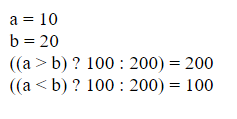#### Mashiane

##### Expert
Do While Loop

B4X:
``````Dim count As Int = 0

write("Starting Loop ")

Do While (count < 10)
write(\$"Current Count : \${count}<br />"\$)
count = count + 1
Loop

write("Loop stopped!")``````
Output: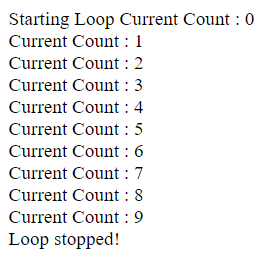Last edited:

#### Mashiane

##### Expert
Select Case / JS Switch.

B4X:
``````Dim grade As String = "A"

write("Entering switch block<br />")

Case "A"
write("Good job<br />")
Case "B"
write("Pretty good<br />")
Case "C"
write("Passed<br />")
Case "D"
write("Not so good<br />")
Case "F"
write("Failed<br />")
Case Else
End Select
write("Exiting switch block")``````
Output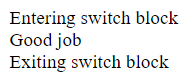•joulongleu

#### Mashiane

##### Expert
If statement

B4X:
``````Dim book As String = "maths"
Dim linebreak As String = "<br />"

write("book = ")
write(book)
write(linebreak)
'
If book = "history" Then
write("<b>History Book</b>")
else if book = "maths" Then
write("<b>Maths Book</b>")
else if book = "economics" Then
write("<b>Economics Book</b>")
Else
write("<b>Unknown Book</b>")
End If``````

Output•joulongleu

#### Mashiane

##### Expert
Do Until Loop

B4X:
``````write("Starting Do Loop ")

Do Until (count = 10)
write(\$"Current Count : \${count}<br />"\$)
count = count + 1
Loop

write("Loop stopped!")``````

Output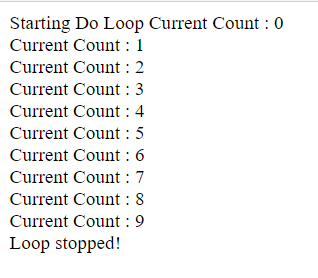•joulongleu